# How to Add Numbers in Microsoft Excel

It’s simple to add numbers with Microsoft Excel. You just have to make a simple formula to accomplish this.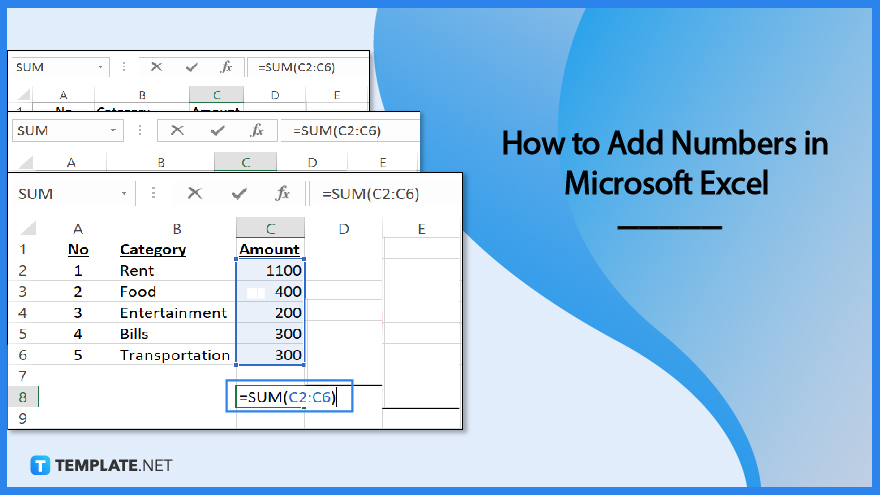## How to Add Numbers in Microsoft Excel

When adding numbers in Microsoft Excel, you must keep in mind that all formulas should start with the equal symbol (=), and you can use the formula bar to create them.

• ### Step 1: Adding Two or More Numbers in a Cell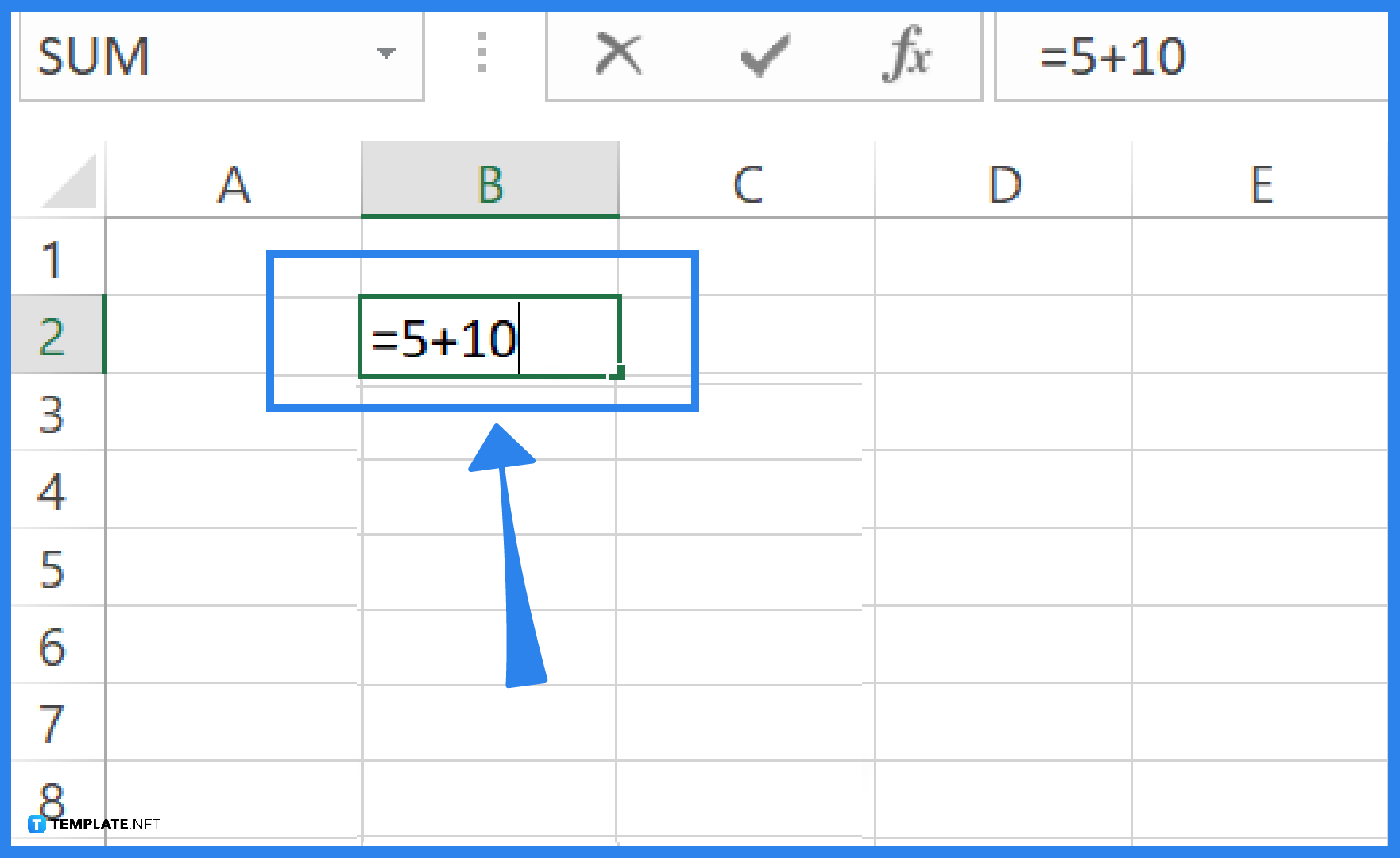If you are adding two or more numbers in a cell, click any blank cell, and input the equal sign (=) first. After that, type a few numbers separated by a plus sign (+). For example, =5+10. Then, press RETURN to get the result. As for the example, you will get 15 as a result.• ### Step 2: Adding Numbers Using Cell References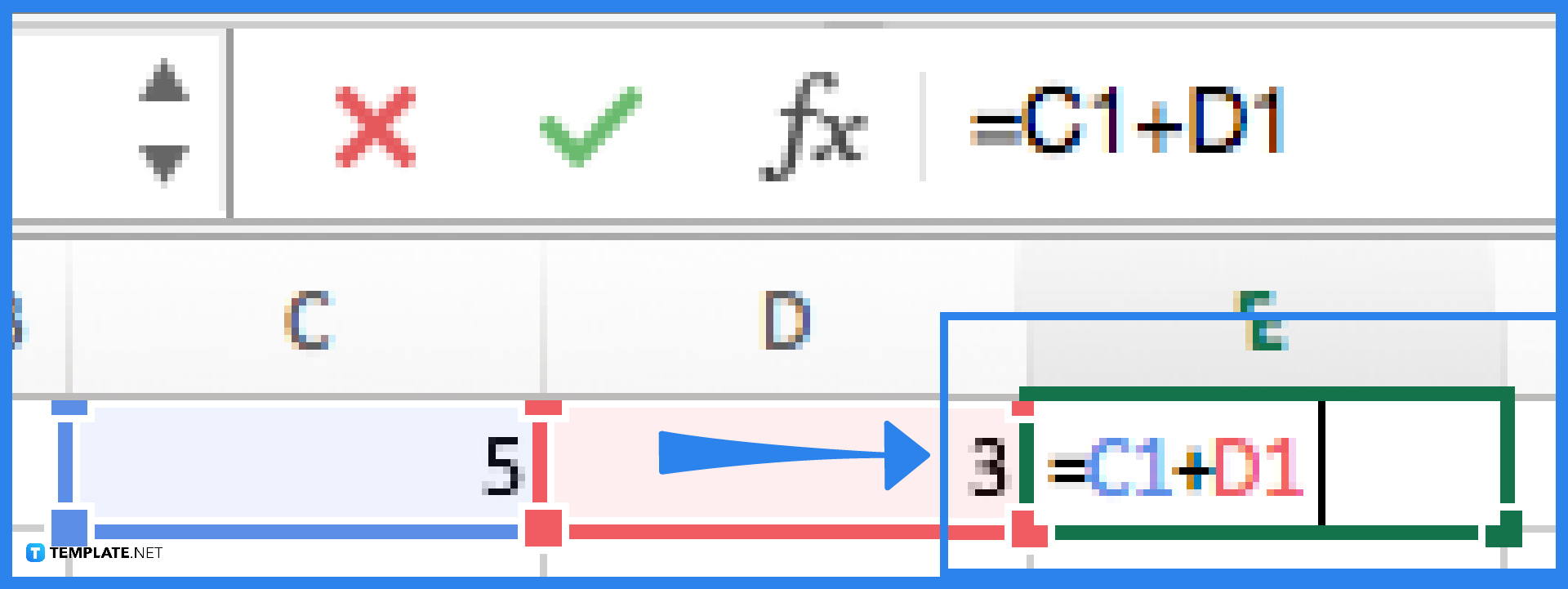Type the numbers in different cells. You can, for example, type 5 in cell C1 and then another number, such as 3, in cell D1. In another blank cell, such as E1, type an equal sign (=) to start the formula. After the equal sign, type the cell references of the numbers you want to add. In the example, you would type the formula as =C1+D1. After that, press RETURN to get the result.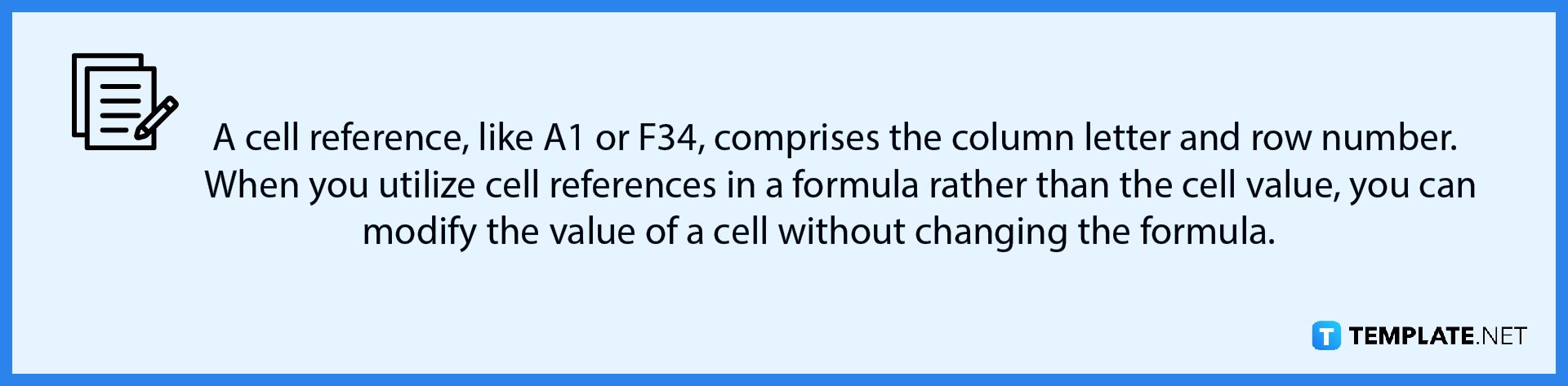• ### Step 3: Adding Numbers Using AutoSumTo use the AutoSum feature, click on the blank cell next to the section where your numbers are located. For example, if your numbers are from C2 to C6, then you must click on C7. After that, select the Home tab from the ribbon. Then click the AutoSum icon in the Editing box on the right. Your numeric range will be automatically selected by Excel and highlighted. Press Enter on your keyboard to get the sum of these numbers.

• ### Step 4: Adding Numbers Using The Sum Function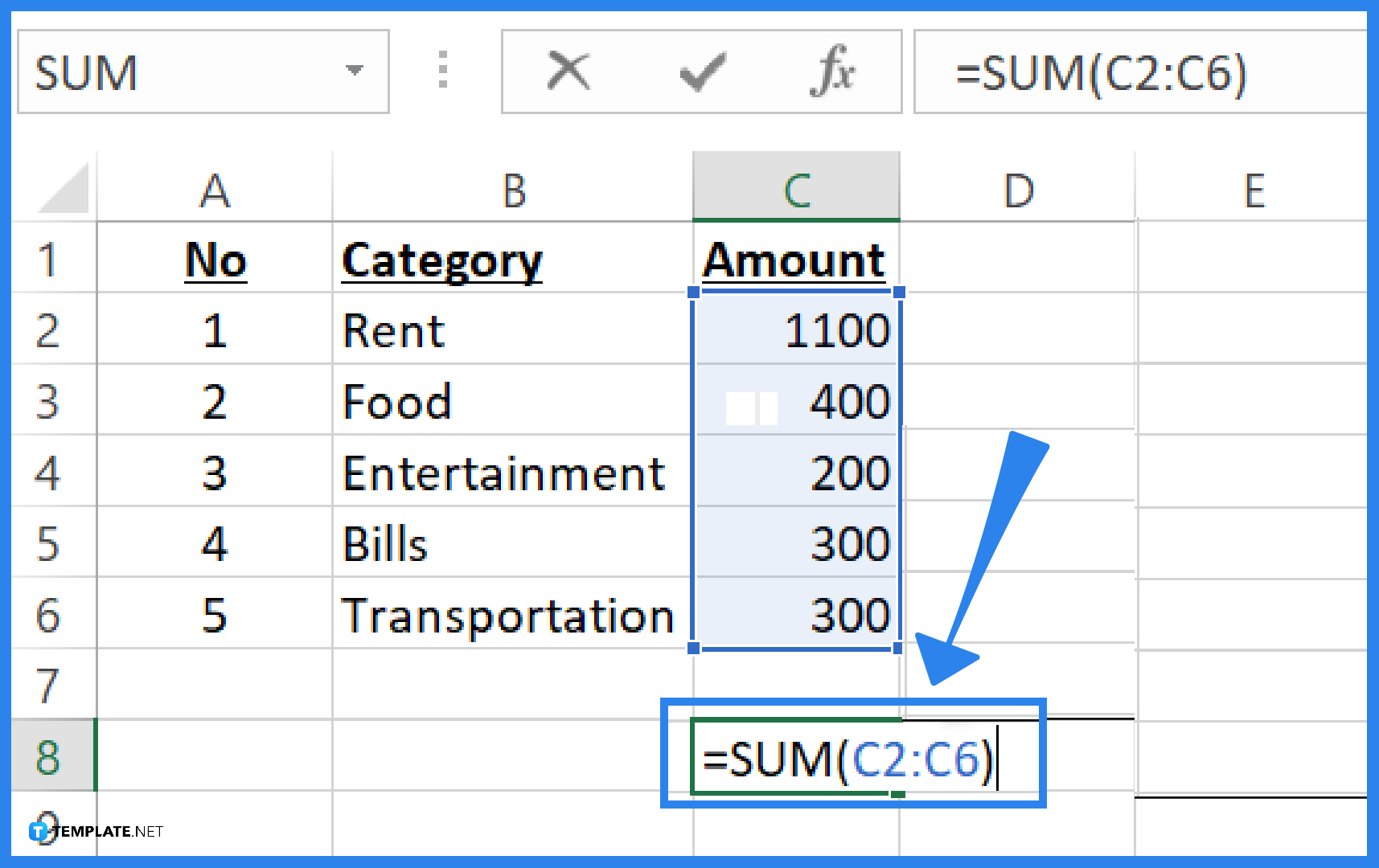Click on the cell in which you want to display the sum. Type an equal sign followed by the word SUM, like this: =SUM. Then, type an opening parenthesis, select the range of cells you want to add, and type the closing parenthesis. After that, press Enter or Return to get the sum.

## FAQs

### What is the most common way to add numbers in Microsoft Excel?

The SUM function is the most popular way to add numbers in Microsoft Excel. The SUM function is designed to return the sum of the values you supply, whether they are individual values, ranges, cell references, or a combination of all three. It is possible to use up to 255 individual references with it.

### Is it possible to get the sum of dates in Microsoft Excel?

Yes. In Microsoft Excel, you can add days, months, years, or a combination of the three to a date. You can also use the EDATE function to add dates.

### How about getting the sum of time in Microsoft Excel?

Microsoft Excel can compute the sum of time. The easiest way to get the total amount of time is by using the AutoSum function.